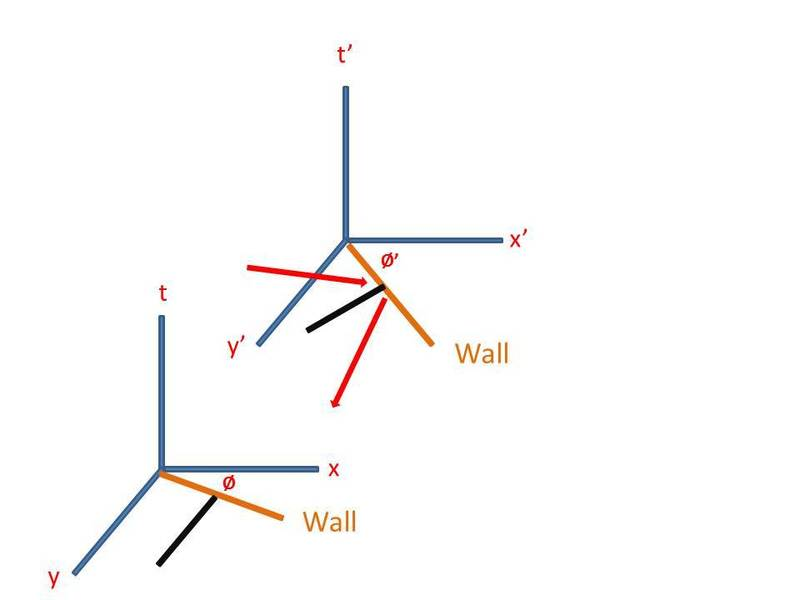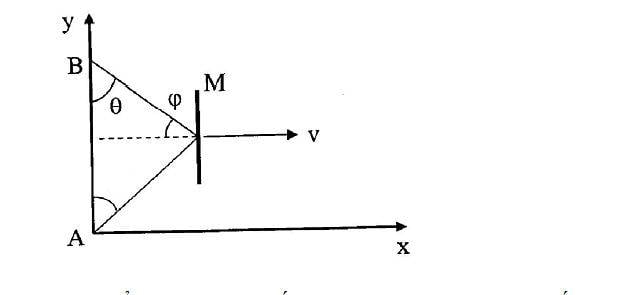# Particle hitting an inclined wall viewed in different frames

## Homework Statement

Consider an inertial frame S with coordinates ##x^μ = (t, x, y ,z)##, and a frame S' with coordinates ##x^{μ'}## related to S by a boost with velocity parameter v along the y-axis. Imagine we have a wall at rest in S', lying along the line x' = -y'. From the point of view of S, what is the relationship between the incident angle of a particle hitting the wall (traveling in the x-y plane) and the reflected angle? What about the velocity before and after?

## Homework Equations

Lorentz Transformations
##θ_i = incident~ angle, ~~~θ_f = reflected~ angle##
##γ = Lorentz~ factor##

## The Attempt at a Solution

We know that ##θ_i' = θ_f'##. Since S' is moving along the y-axis, the wall would appear to be contracted only in the y direction. Suppose the wall has length L, then the y component of the wall as viewed in S is
##y = \frac{L~sin(φ')}{γ}##
The x component of the wall is the same as in the S' frame, so the angle ##φ## that the wall makes with respect to the x axis is
##φ = tan^{-1}(\frac{\frac{L~sin(φ')}{γ}}{L~cos(φ')}) = tan^{-1}(\frac{tan(φ')}{γ})##
This angle ##φ## will be smaller compared to ##φ'##, their difference ##φ' - φ## is the increase in angle the observer in S will see for the incident angle (decrease for the reflected angle), so the incident and reflected angles as seen in S are
##θ_i = θ_i' + (φ' - φ). ~~~ θ_f = θ_f' - (φ' - φ)##
The velocity before and after in frame S are just related by Lorentz transformations to the velocity before and after in the frame S'.

I'm not sure if my argument for the incident and reflected angle is correct, can anyone help check my work? Thanks.##θ_i = θ_i' + (φ' - φ). ~~~ θ_f = θ_f' - (φ' - φ)##
Why ?
First, you try find ##θ_i&θ_f## in a simple case if the wall only moves along in x-axis, the face of wall parallel with y-axis. Then φ' - φ=0 so ##θ_i = θ_i'## but in this case ##θ_i = θ_i'## seems incorect.View attachment 102709[/QUOTE]

Last edited:
##θ_i = θ_i' + (φ' - φ). ~~~ θ_f = θ_f' - (φ' - φ)##
Why ?
First, you try find ##θ_i&θ_f## in a simple case if the wall only moves along in x-axis, the face of wall parallel with y-axis. Then φ' - φ=0 so ##θ_i = θ_i'## but in this case ##θ_i = θ_i'## seems incorect.View attachment 102709
[/QUOTE]
Because the length contraction is along the y' axis and by taking the angle as viewed in S, that angle would be smaller compared to the angle that the wall makes with the x axis in S. But then the decrease in angle for the wall is the increase for the incident angle (with respect to the normal of the wall), vice versa for the reflected angle.

##θ_i = θ_i' + (φ' - φ). ~~~ θ_f = θ_f' - (φ' - φ)##
Why ?
First, you try find ##θ_i&θ_f## in a simple case if the wall only moves along in x-axis, the face of wall parallel with y-axis. Then φ' - φ=0 so ##θ_i = θ_i'## but in this case ##θ_i = θ_i'## seems incorect.View attachment 102709
[/QUOTE]
By assuming that the wall is parallel to the y-axis then I think the incident and reflected angles shouldn't change, but in this case of course the wall is inclined.

incident and reflected angles must change because for incident angel,we see in the S frame, incident angle moves with velocity v along Ox , but for reflected angle it moves contrariwise with velocity -v. So the Lorentz Transformations will be different.

##θ_i = θ_i' + (φ' - φ). ~~~ θ_f = θ_f' - (φ' - φ)##
Why ?
First, you try find ##θ_i&θ_f## in a simple case if the wall only moves along in x-axis, the face of wall parallel with y-axis. Then φ' - φ=0 so ##θ_i = θ_i'## but in this case ##θ_i = θ_i'## seems incorect.View attachment 102709
[/QUOTE]
Sorry for the long gap before the reply. So in this case, I can suppose that the incident velocity is u' and decompose its components by the velocity transformation law

##u_x = \frac{ u'cosθ'_i + v }{ 1 + \frac{u'vcosθ'_i}{c^2} } ~~~, ~~~u_y = \frac{ u'sinθ'_i }{ γ(1 + \frac{u'vsinθ'_i}{c^2}) } ##

The components of the reflected velocity would just be the same except for a negative sign in the x component based on your diagram.

##u_x = \frac{ -u'cosθ'_f + v }{ 1 - \frac{u'vcosθ'_f}{c^2} } ~~~, ~~~u_y = \frac{ u'sinθ'_f }{ γ(1 + \frac{u'vsinθ'_f}{c^2}) } ##

But how do I relate the incident and reflected angle as seen in S?